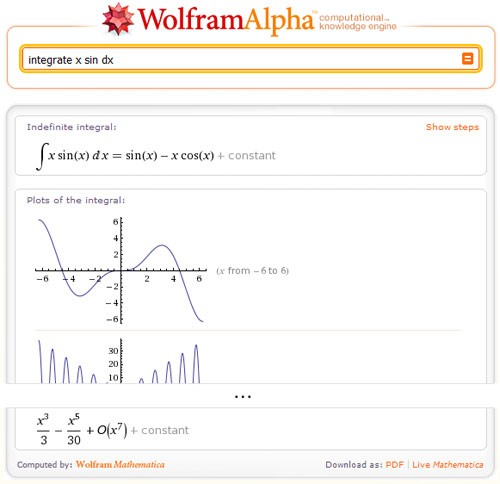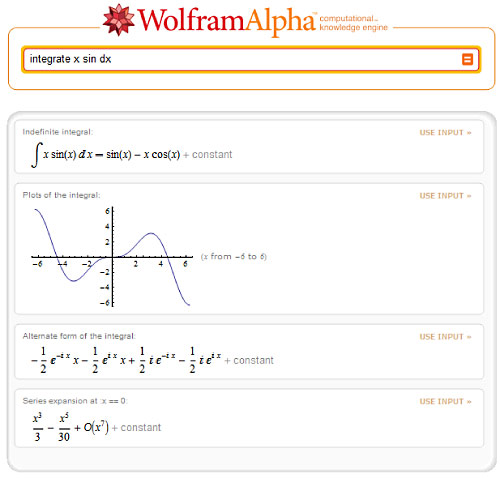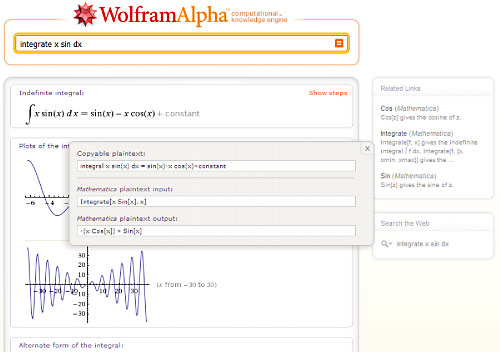# Wolfram|Alpha Is Launching: Made Possible by Mathematica

(This post was originally published on the Wolfram|Alpha Blog.)

Starting later today, we’ll be launching Wolfram|Alpha (you can see the proceedings on a live webcast).

This is a proud moment for us and for the whole Mathematica community. (We hope the launch goes well!)

Wolfram|Alpha defines a new direction in computing—that would have simply not have been possible without Mathematica, and that in time will add some remarkable new dimensions to Mathematica itself.

In terms of technology, Wolfram|Alpha is a uniquely complex software system, which has been entirely developed and deployed with Mathematica and Mathematica technologies.

We are still at the beginning of Wolfram|Alpha as a long-term project, but already it contains nearly 6 million lines of Mathematica code—all, of course, authored and maintained in Wolfram Workbench.

When we launch Wolfram|Alpha this weekend, it will be running Mathematica on about 10,000 processor cores, using gridMathematica-based parallelism. And every single query that comes into the system will be served with webMathematica.

If you watch the live webcast tonight, you’ll see lots of real-time system analysis, logging, and visualization—all written in Mathematica.

I’ve been thinking about building Wolfram|Alpha for a very long time.

But one of the most important things that’s made it possible now is the state of Mathematica today.

We’ve always thought that Mathematica was an increasingly development environment. But what we’ve done with the development of Wolfram|Alpha in the past few years serves as living proof.

The Mathematica Computable Data Initiative was the testbed for the large-scale data curation system that we’ve set up for Wolfram|Alpha. And this curation system itself relies on many features of Mathematica. We’re importing data in almost every conceivable format using Mathematica’s import capabilities. We’re combining and analyzing data using Mathematica’s data, statistics and visualization capabilities. Our human curation and expert review process is done using Mathematica symbolic documents, with extensive dynamic interface capabilities. And our whole central data repository system is written in Mathematica, with symbolic representations of data and its relationships, and efficient underlying use of DatabaseLink for relevant large and real-time datasets.

Already in Mathematica 7 we’ve integrated many kinds of data seamlessly into the Mathematica language. And within Mathematica it’s possible to do arbitrarily flexible large-scale computations with the data. Wolfram|Alpha adds tremendous ease of use for small-scale computations—as well as introducing a large number of new types of data, which can potentially be accessed for large-scale computation in Mathematica in the future.

Beyond curated data, another important aspect of Wolfram|Alpha is its ability to actually do computations. In building Wolfram|Alpha, we’ve been systematically implementing all the methods and models of science and other areas whether quantitative approaches can be used. This is of course an immense undertaking, and without Mathematica it would have been impossible.

The coherent symbolic structure of Mathematica is crucial to constructing the frameworks that make the programming even vaguely conceivable. And in the actual implements of so many models and methods, we’re using practically every corner of Mathematica‘s huge web of algorithmic capabilities.

Often there may be some simple approximation that could be implemented just as a formula. But because we have Mathematica, we can do the real computation—and get real state-of-the-art results, solving differential equations, performing global optimizations, and doing whatever sophisticated math or computations are needed.

One of the highly visible features of Wolfram|Alpha is its ability to understand free-form linguistic input. And this too would have been impossible without Mathematica.

There are many parts to Wolfram|Alpha’s linguistic processing system. But one common theme is the deep use of symbolic programming and Mathematica‘s unique symbolic pattern-matching capabilities. And here again the immediate access to efficient algorithms is also crucial—whether it be for string processing, discrete math or something else. (Who knew that parsing nested sequences of |’s is best done with efficient linear programming?)

Yet another central aspect of Wolfram|Alpha is its automated presentation of results. And here again, many parts of Mathematica play crucial roles.

Every output from Wolfram|Alpha is constructed by the Wolfram|Alpha server as a Mathematica notebook. The ability to automatically typeset tables, math and collections of graphics of unknown sizes and shapes is crucial.

And the automatic computational aesthetics built into Mathematica are what have made it practical to implement thousands of different kinds of visualizations in Wolfram|Alpha.

If one looks at the internal code of Wolfram|Alpha, it’s amazing how diverse the uses of Mathematica are. Two principles bring it all together.

The first is the symbolic character of Mathematica—which makes it possible to implement so many diverse kinds of knowledge in a unified way, and have them successfully interact with each other.

The second is automation—the idea that functions in Mathematica should be as automated as possible—automatically selecting algorithms, choosing visualizations, working out import formats, and so on. Because automation is what makes it feasible to create a robust system of the magnitude and functionality of Wolfram|Alpha with manageable resources.

And even beyond the actual user-visible functionality of Wolfram|Alpha, Mathematica has been crucial in the project.

All of the many automated testing systems for Wolfram|Alpha are written in Mathematica—and rely on such diverse capabilities as parallel computation, symbolic structure comparison, and bitmap image manipulation.

Mathematica has been extensively used in meta-analysis of the code base and of the development process itself. And for example the ability to easily create complex interactive algorithmic diagrams—say to represent stages in linguistic processing—has been crucial.

So Mathematica is what’s inside Wolfram|Alpha—and what’s made Wolfram|Alpha possible.

But how will Wolfram|Alpha interact with Mathematica?

There are several ways.

First, at the bottom of each Wolfram|Alpha output page, there’s a button that says “Download Live Mathematica”.What that does is to download the results on the page as a Mathematica notebook.The Mathematica notebook has many immediate advantages over Wolfram|Alpha’s standard web presentation.

First, the graphics are resolution-independent and resizable. And 3D graphics can immediately be zoomed and rotated.

But what’s more important is that you can take the output from Wolfram|Alpha and actually compute with it in Mathematica.

And you can also click the Use Input button on particular Wolfram|Alpha output pods in the notebook, and get Mathematica input that would generate the basic output in the pod.

In fact, in a sense, Wolfram|Alpha is the ultimate “discovery interface” for Mathematica. If you want to know what how to refer to something in CountryData, just enter it in free-form linguistics in Wolfram|Alpha, and Wolfram|Alpha will show you the precise Mathematica syntax for what you want.

One way to see the Mathematica code is to get the Live Mathematica Notebook.

But if you just want to know the Mathematica code for a particular Wolfram|Alpha output pod, you can click on the pod and look at the popup.

So long as there’s a simple representation of the pod computation in Mathematica, there’ll be a “Mathematica plaintext input” field in the popup—that you can copy and paste anywhere. (Notice also the links to Mathematica function information in the right sidebar.)So if you ever forget how to specify a computation in Mathematica, you can just go to Wolfram|Alpha and enter the computation in free form, using any kind of natural notation or language.

Needless to say, I’m sure this capability will be important when people are first learning Mathematica—and it’s one of the things future versions of Mathematica will be able to call on Wolfram|Alpha to do.

In a sense, Mathematica and Wolfram|Alpha take opposite positions in the representation of computation.

Wolfram|Alpha is set up to have short one-off inputs, that can sloppily mix all sorts of notations with fragments of natural language. And Wolfram|Alpha then works by using its linguistic processing capabilities to figure out what the most likely meaning of the input is.

Mathematica, on the other hand, is set up to be a precise, coherent, consistent system, where inputs follow clear general principles—and can combined in completely arbitrary ways, to build up programs of arbitrary complexity, like the nearly 6 million lines of Wolfram|Alpha’s own code base.

Over the next few years, I suspect some of the most dramatic and unexpected advances will come from combining the Wolfram|Alpha and the Mathematica views of computation.

Wolfram|Alpha will soon support an API, and Mathematica will probably be its richest client.

But as we imagine the future of Mathematica symbolic notebook interfaces and local computation, interacting with large-scale central Wolfram|Alpha servers and the breadth of Wolfram|Alpha’s capabilities, the possibilities are quite spectacular.

Wolfram|Alpha introduces a concept of knowledge-based computing—that builds on Mathematica, and then provides remarkable new power for Mathematica itself.

If the past is a guide, it’ll be quite a few years before we understand the full implications of all these possibilities.

But as of now—today—it’s exciting to see just what we have been able to build in Wolfram|Alpha with Mathematica.

And quite soon, well over 10,000 Mathematicas will be running on our compute servers to launch what’s probably the most complex and computation intensive website ever.

And begin the next phase in the evolution of computing—all made possible by Mathematica.